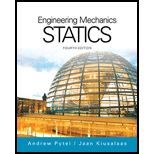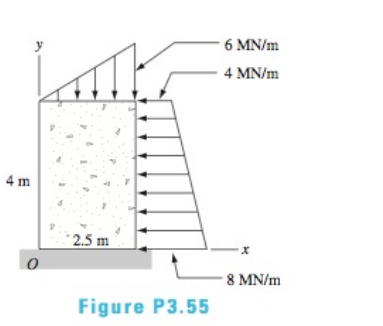# The concrete pier is subjected to soil pressure that causes the line loads shown. Determine the resultant of the loading and find the y-coordinate of the point where the resultant crosses the y -axis### International Edition---engineerin...

4th Edition
Andrew Pytel And Jaan Kiusalaas
Publisher: CENGAGE L
ISBN: 9781305501607

#### Solutions

Chapter
Section### International Edition---engineerin...

4th Edition
Andrew Pytel And Jaan Kiusalaas
Publisher: CENGAGE L
ISBN: 9781305501607
Chapter 3, Problem 3.55P
Textbook Problem
184 views

## The concrete pier is subjected to soil pressure that causes the line loads shown. Determine the resultant of the loading and find the y-coordinate of the point where the resultant crosses the y-axisTo determine

Resultant force and y -coordinate of the point where resultant crosses the y -axis.

### Explanation of Solution

Given Information:

The distributed load is shown in following figure

:

Calculation:

Consider the following figure,

The resultant of horizontal force is

:

Fx=( 8+4)×42=24 MNact at y=( 2×4+8)×43(4+8)=( 2×4+8)×43(4+8)=1.78 m

The resultant of vertical force is

:

Fy=6×2.52=7.5 MNact at x=23×2.5=1

### Still sussing out bartleby?

Check out a sample textbook solution.

See a sample solution

#### The Solution to Your Study Problems

Bartleby provides explanations to thousands of textbook problems written by our experts, many with advanced degrees!

Get Started

Find more solutions based on key concepts
Explain how to use a push stick.

Precision Machining Technology (MindTap Course List)

What is a knowledge repository?

Fundamentals of Information Systems

Give examples of properties that engineers consider when selecting a material for a product or an application.

Engineering Fundamentals: An Introduction to Engineering (MindTap Course List)

What does communications software do? (457)

Enhanced Discovering Computers 2017 (Shelly Cashman Series) (MindTap Course List)

How does an injector-type mixing chamber work?

Welding: Principles and Applications (MindTap Course List)

If your motherboard supports ECC DDR3 memory, can you substitute non-ECC DDR3 memory?

A+ Guide to Hardware (Standalone Book) (MindTap Course List)